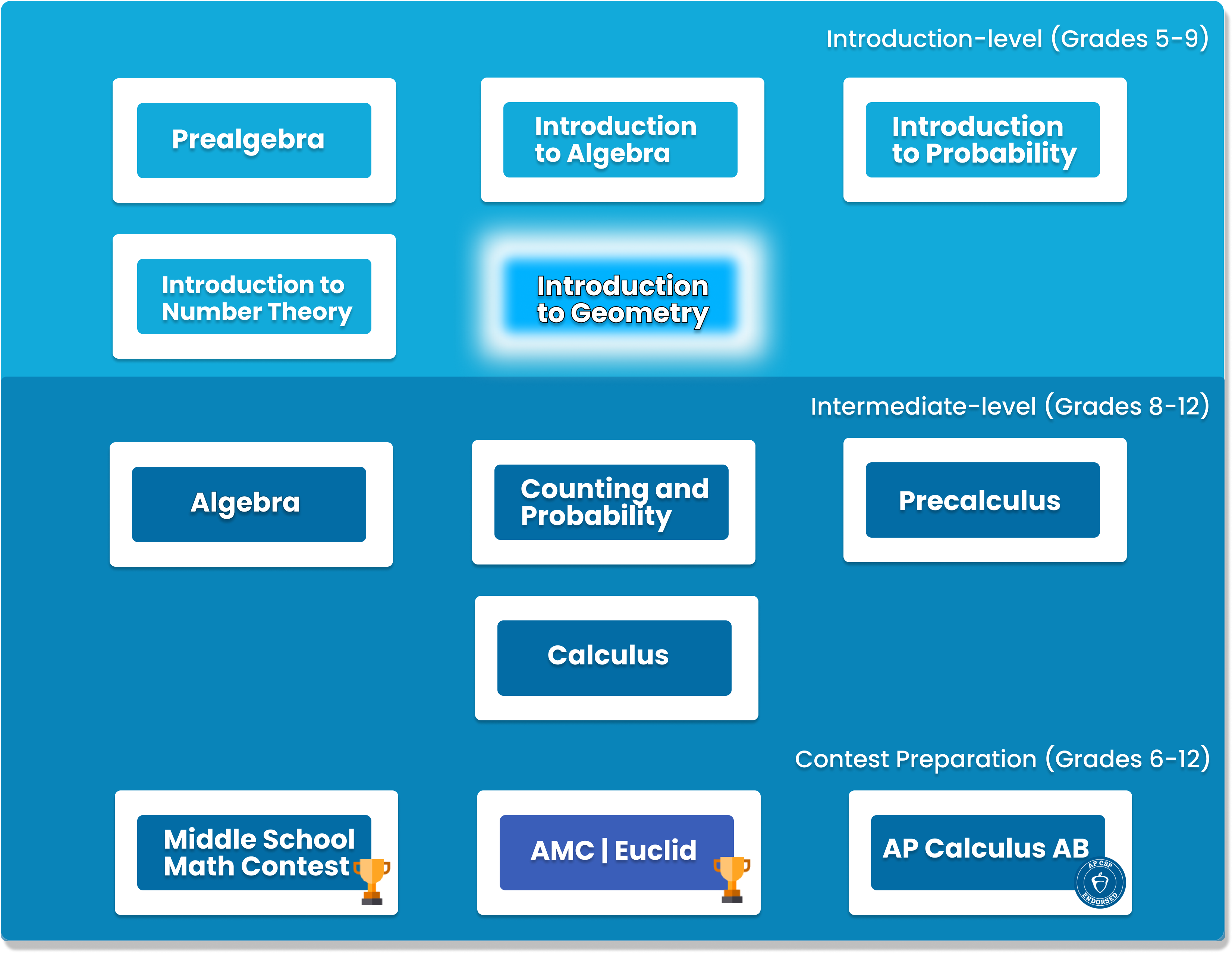# Introduction to Geometry

Students in grades 6-10 will build a solid understanding of more challenging geometry concepts in this course. It is designed to expand on high school geometry topics##### Who is this course for
This Course is for Grade 5-Grade 9 Students who want to advance their knowledge in Geometry.
This course typically takes 3-6 months to complete. This depends on the student's experience and how fast they can master the concepts and the knowledge. Our instructors move at the pace of the student, it may take extra time for some students to reinforce what they have learned.
##### Curriculum
###### Chapter 1: What’s in a NameNotation used in geometry, points, lines, and planes
###### Chapter 2: AnglesAngles, parallel lines, angles in a triangle, exterior angles
###### Chapter 3: Congruent TrianglesLearn to determine if two triangles are congruent, study properties of isosceles and equilateral triangles
###### Chapter 4: Perimeter and areaPerimeter, area
###### Chapter 5: Similar trianglesLearn to determine if two triangles are similar, properties of similar triangles
###### Chapter 6: Right TrianglesPythagorean Theorem
###### Chapter 7: Special Parts of a trianglePerpendicular and angle bisectors of a triangle, medians, altitudes
###### Chapter 8: QuadrilateralsTrapezoids, parallelograms, Rhombi, rectangles, squares
###### Chapter 9: PolygonsAngles and area of polygons
###### Chapter 10: Geometric InequalitiesSides and angles of a triangle, the triangle inequality
###### Chapter 11: CirclesArc length, areas
###### Chapter 12: Circles and anglesInscribed angles, tangents of circles
###### Chapter 13: Three-Dimensional GeometryPlanes, Prisms, Pyramids
###### Chapter 14: Curved SurfacesCylinders, Cones, Spheres
###### Chapter 15: The more things changeTranslation, rotations, reflections, dilation
###### Chapter 16: Analytic GeometryGeometry in coordinate plane, lines, Circles, distance between a point and a line.
###### Chapter 17: Introduction to trigonometryTrigonometry ratios, law of sines, law of cosines
###### Chapter 18: Problem Solving Strategies in geometryTechniques to solve geometry problems, including adding auxiliary lines

### You Might Also Be Interested In Our Elective Courses##### Python Level 1
AGES 10 - 15

Designed for beginners, this level is designed to teach the basic fundamentals and design principles of Python, with the help of Turtle graphics and PyGame. This includes: variables, conditional statements, loop basics, and functions.

These concepts are transferable to any other programming language. Throughout the way, students will create projects in order to apply the concepts they have learned, and to solidify their knowledge.##### Python Level 2
AGES 10 - 15

Level 2 dives deeper into the basics of Python for a more thorough understanding and introduces advanced topics. Students will build on knowledge from Level 1 and work with data structures, advanced loops, algorithms, and object-oriented programming, and create games based on what they learn.

Students will complete the course with a solid understanding of Python fundamentals.##### Java Level 1
AGES 10 - 15

This beginner-friendly course serves as an introduction to the Java programming language. Students will learn the fundamentals of Java along with core computer science concepts.